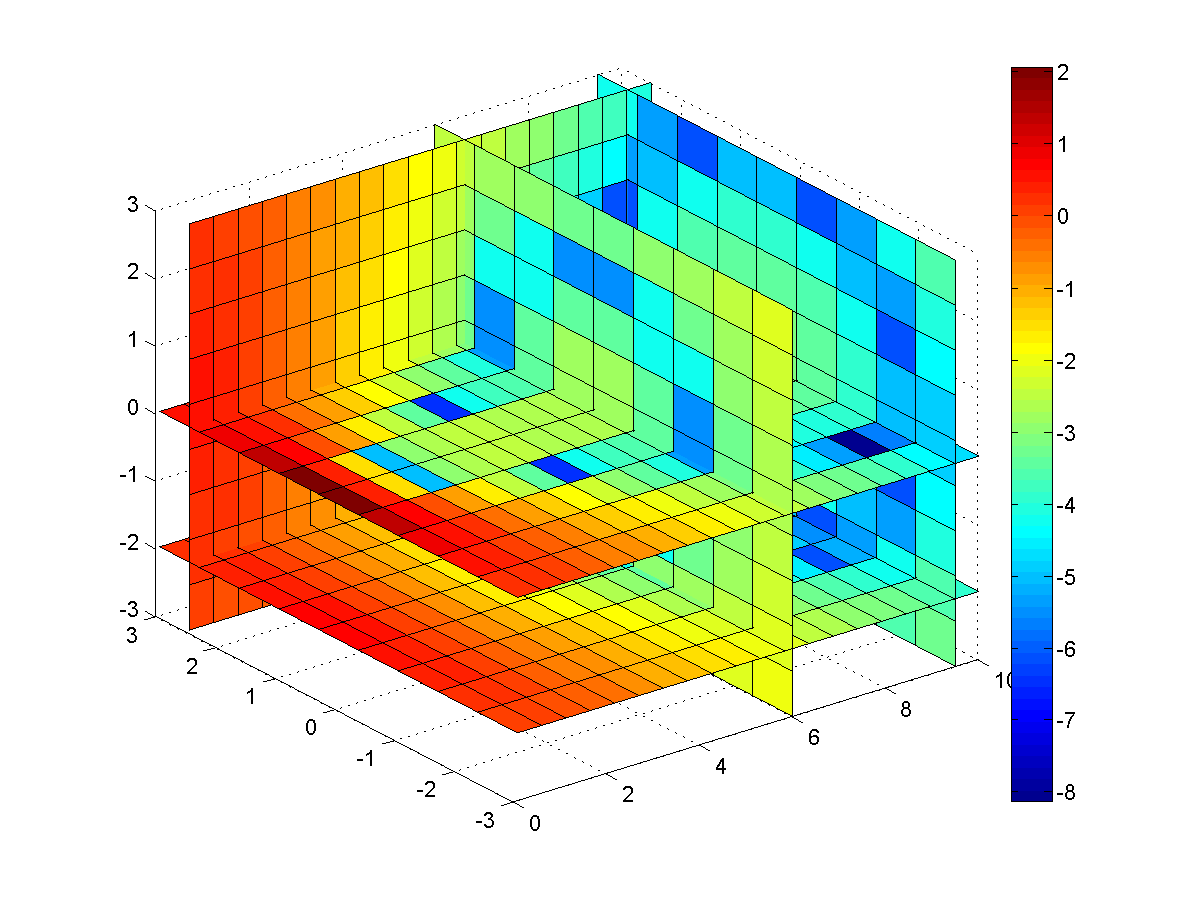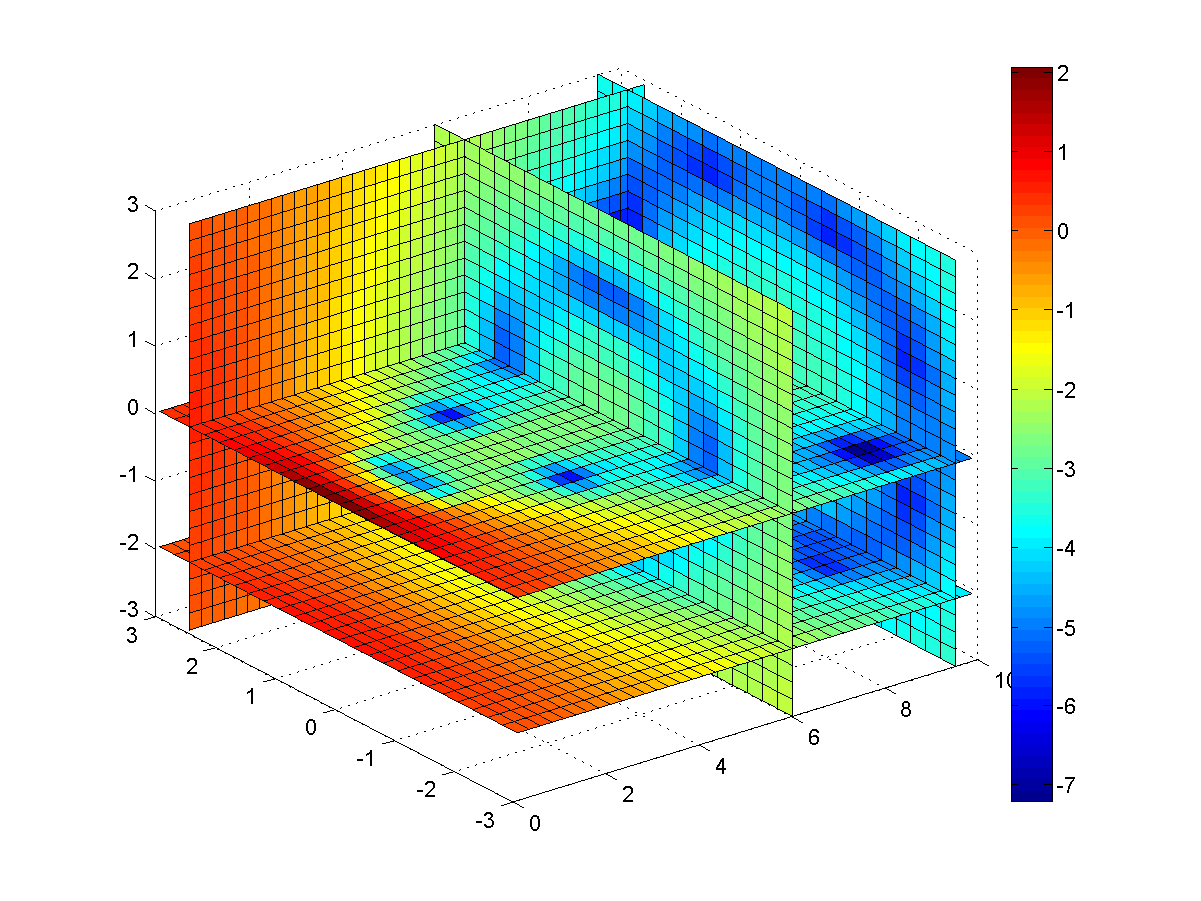## 9-4 嚙踝蕭嚙踝蕭嚙踝蕭嚙踝蕭嚙踝蕭嚙踝蕭嚙踝蕭嚙踝蕭嚙踝蕭嚙踝蕭嚙踝蕭嚙踝蕭

MATLAB 的 interp3 可進行 3 維格子點的內插，其一般格式為

vi = interp3(x, y, z, v, xi, yi, zi, method)

• 'nearest'：鄰近點內插
• 'linear'：線性內插
• 'cubic'：三次內插
• 'spline'：Spline 內插法

Example 1: 09-內插法/slice01.m[x, y, z, v] = flow(10); slice(x, y, z, v, [6 9.5], 2.5, [-2 0]); colorbar; % 顯示顏色與函數值的對照表 size(x) ans = 10 20 10Hint
• 在MATLAB常用的1維輸入的函數是 humps，常用來測試曲線的零點或是極值。
• 常用的2維輸入的函數是 peaks，常用來測試曲面圖或是等高線。
• 而常用的三維輸入的函數就是 flow，常用來測試切片圖或三維內插。

Example 2: 09-內插法/interp301.m[x, y, z, v] = flow(10); [xi, yi, zi] = meshgrid(.1:.25:10, -3:.25:3, -3:.25:3); vi = interp3(x, y, z, v, xi, yi, zi); slice(xi, yi, zi, vi, [6 9.5], 2.5, [-2 0]); % 產生切片圖 colorbar; % 顯示顏色與函數值的對照表 size(xi) ans = 25 40 25Hint

MATLAB程式設計：進階篇